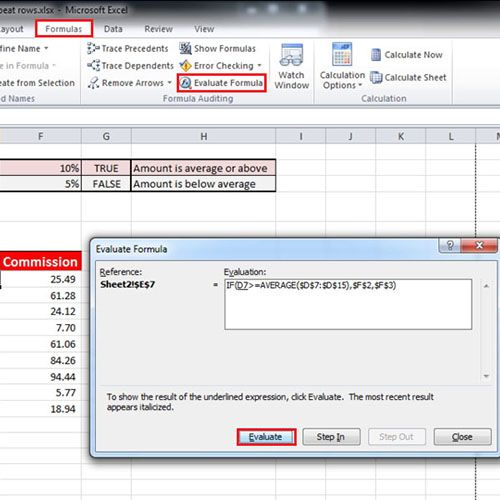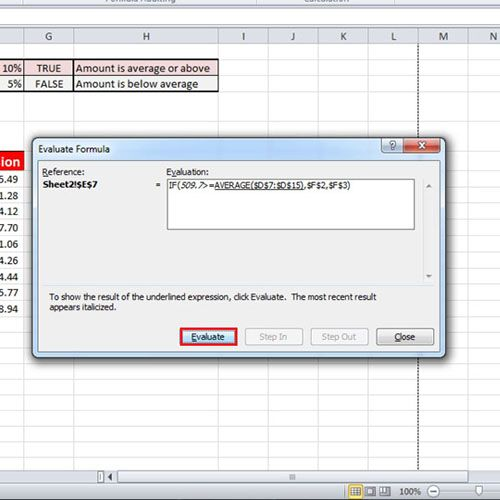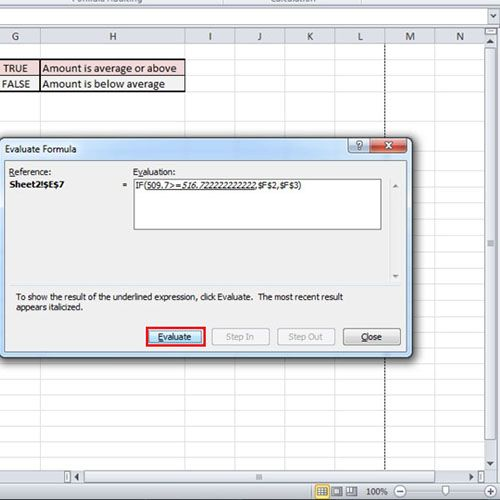## How to Evaluate Formula in Excel

Microsoft Excel is a detailed software that can be used as a multiple analysis tool. It gives simple options that can help you manage and manipulate data to derive desired or actual results. It is important to understand the workings of this software to be able to use it effectively. Working with formulas in Excel is quite easy and very helpful. Huge amounts of data can be worked with using these formulas. To learn how to evaluate formulas in Excel, follow this step by step tutorial.

Step # 1 – Evaluate Formula in Formula Auditing

Over here, we have the Sales per person and the commission rate, for which we have applied a formula. Let’s click on the first cell of the, “Commission Rate” column and go to the “Formulas” tab. Now, in the “Formula Auditing” section, click on the “Evaluate Formula” in Excel. The “B5” is underlined because it is under evaluation. Now click on the “Evaluate” button and it will show what the selected cell contains. Now the next argument is underlined, click on the “Evaluate” button again and it will show the average of the column.Step # 2 – Click on Evaluate

If you press the “Evaluate” button again, it will tell whether the selected argument is False or not. Over here, this formula in Excel is supposed to give an answer based on the Sales value.Step # 3 – Conditions applied on Formula

If the value is greater than or equal to the average of the entire sales column, then it is True and a commission rate of 10% will be applied. If it is less than the average, which is the scenario over here, then it will be false and a rate of 5% will be applied.